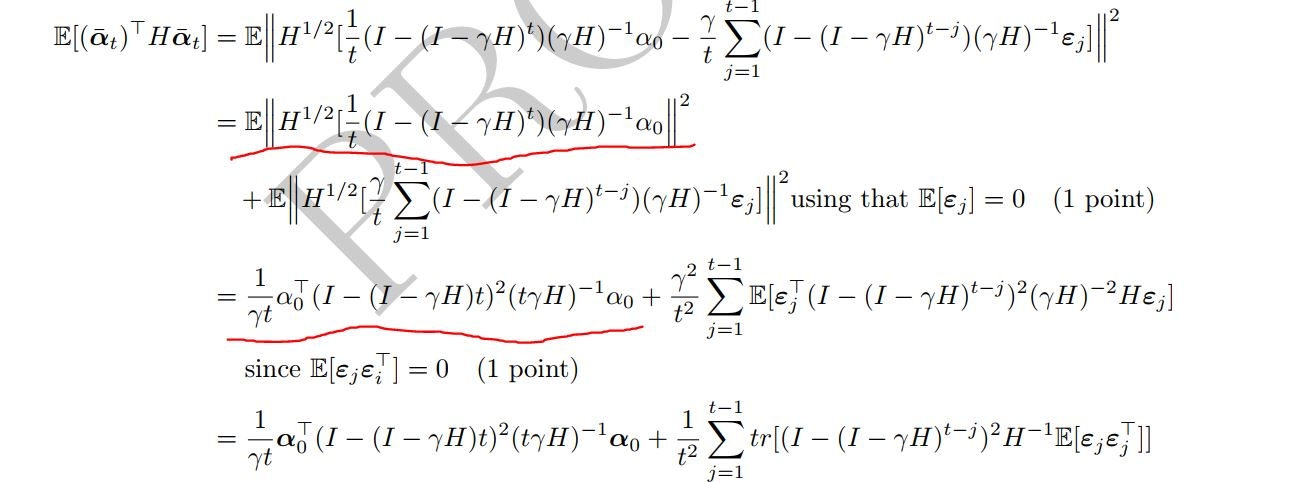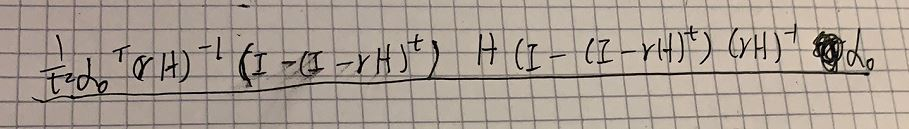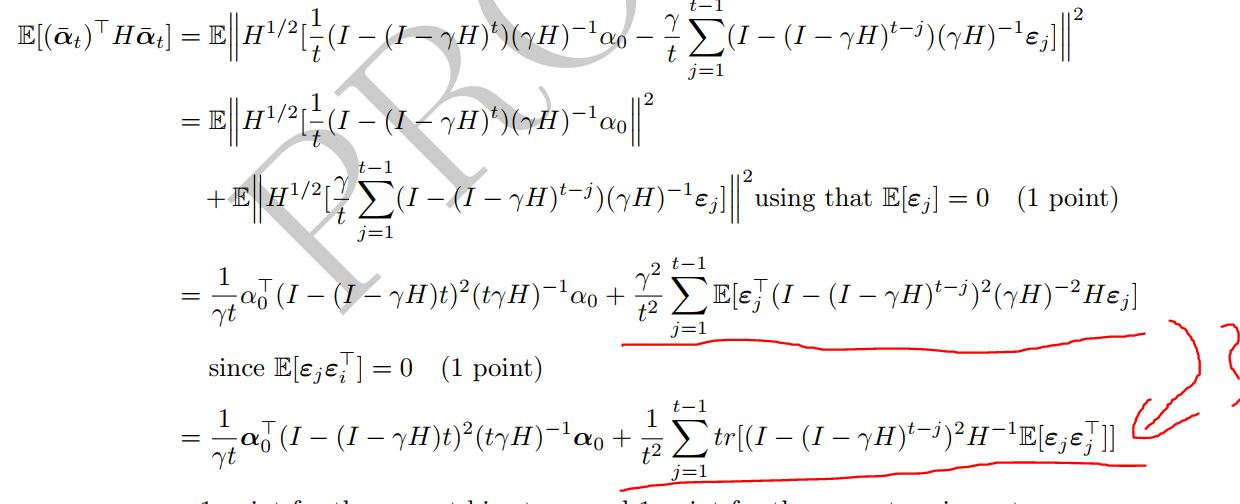### Question for Q34 in exam 2020

Hi,

I have a question for the Q34 in exam 2020. I checked out the solution and found that a change of orders for matrix multiplication is applied between the two underlined steps. Could you please explain why we can change the orders of matrix multiplication in this case? Thank you very much.Without a change of orders, I obtained as follows.Top comment

We didn't change the orders of matrix multiplication. In fact this equality holds true $$H^{-1} (I-(I-\gamma H)^t)H= (I-(I-\gamma H)^t)$$.

Hi,

I have another question. May I ask why the second term in the last line is the trace of the matric product? How can we conclude that? Thank you very much.

This is due to the fact that (when it makes sense to swap order) $$\text{trace}( A B) = \text{trace}(B A)$$ so:
$$\text{trace}( x^\top M x) = \text{trace}( M x x^\top)$$ .

Hi，

It is my fault and I should have made my question clear. How can we transform the product to a trace. (i.e. Why the product is a trace?)@Anonymous said:
Hi，

It is my fault and I should have made my question clear. How can we transform the product to a trace. (i.e. Why the product is a trace?)This is what Scott's comment about by taking $$x=\epsilon_j$$ and $$M=(I-(I-\gamma H)^{t-j})^2H^{-1}$$.